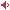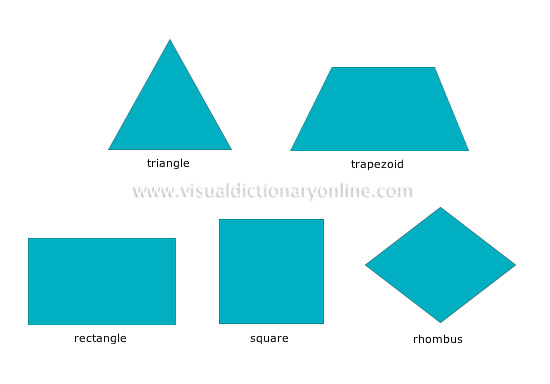# Visual Dictionary Online

Theme

#### polygonsGeometric plane figures with several sides and a number of equal angles.#### trapezoidQuadrilateral with two sides (bases) that are parallel. It is isosceles when it has two sides that are equal and not parallel, and rectangle when two of its sides form a right angle.

#### rectangleQuadrilateral whose opposite sides are equal in length; the sides meet at right angles.

#### squareEquilateral rectangle with four right angles.

#### rhombusEquilateral parallelogram.

#### triangleThree-sided polygon; triangles are scalene (no side is equal to any other) isosceles (two sides equal) or equilateral (all sides equal).